# Use the graph of y f(x) to graph the function g(x) f(x-2)+3Choose the correct graph of g below.yf(x)O A.B.6-4-2-6-2D.4-6

Question

How would you solve this equation to find the correct graph?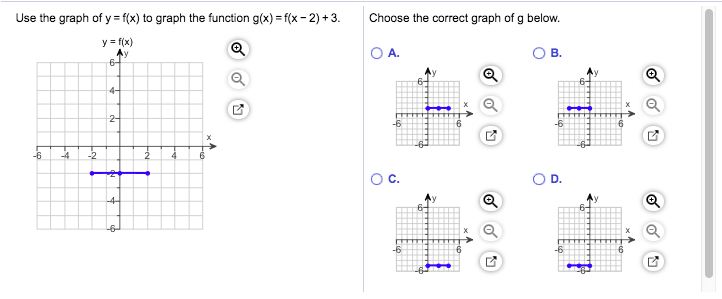help_outlineImage TranscriptioncloseUse the graph of y f(x) to graph the function g(x) f(x-2)+3 Choose the correct graph of g below. yf(x) O A. B. 6- 4- 2- 6 -2 D. 4- 6 fullscreen
check_circle

Step 1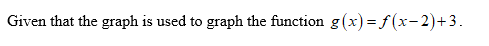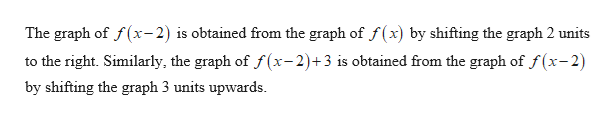help_outlineImage TranscriptioncloseThe graph of f(x-2) is obtained from the graph of f(x) by shifting the graph 2 units to the right. Similarly, the graph of f (x-2)+3 is obtained from the graph of f(x-2) by shifting the graph 3 units upwards. fullscreen
Step 2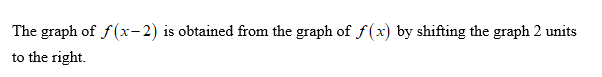Step 3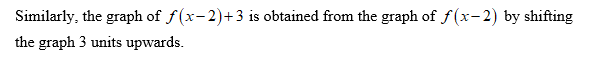...

### Want to see the full answer?

See Solution

#### Want to see this answer and more?

Solutions are written by subject experts who are available 24/7. Questions are typically answered within 1 hour.*

See Solution
*Response times may vary by subject and question.
Tagged in

### Other